# Quiz: Dijkstra’s algorithm¶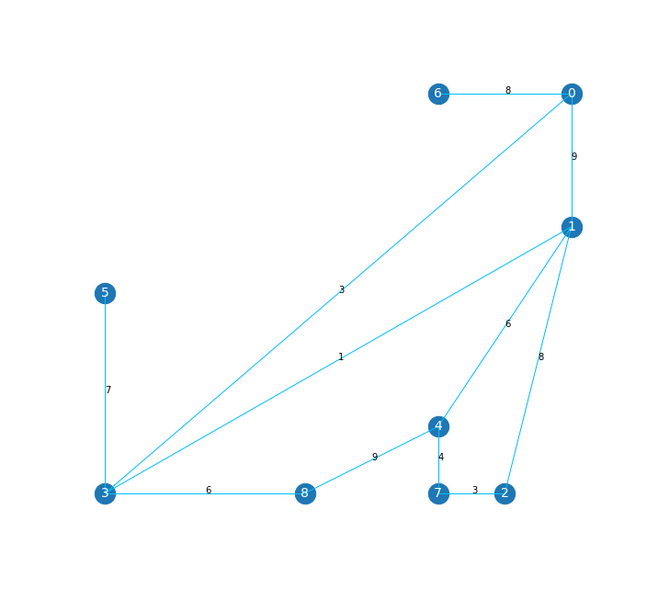Consider the following graph G. It consist of 9 nodes labeled with numbers as well as 12 edges connecting the nodes. Each edge is labeled with its cost. Let 5 be the start node and 7 be the goal node. Run Dijkstra’s algorithm on G. What is the total cost of the path from start to goal?Consider the following graph G. It consist of 9 nodes labeled with numbers as well as 12 edges connecting the nodes. Each edge is labeled with its cost. Let 4 be the start node and 5 be the goal node. Run Dijkstra’s algorithm on G. What is the total cost of the path from start to goal?Consider the following graph G. It consist of 9 nodes labeled with numbers as well as 12 edges connecting the nodes. Each edge is labeled with its cost. Let 2 be the start node and 6 be the goal node. Run Dijkstra’s algorithm on G. What is the total cost of the path from start to goal?Consider the following graph G. It consist of 9 nodes labeled with numbers as well as 12 edges connecting the nodes. Each edge is labeled with its cost. Let 8 be the start node and 2 be the goal node. Run Dijkstra’s algorithm on G. What is the total cost of the path from start to goal?Consider the following graph G. It consist of 9 nodes labeled with numbers as well as 12 edges connecting the nodes. Each edge is labeled with its cost. Let 3 be the start node and 7 be the goal node. Run Dijkstra’s algorithm on G. What is the total cost of the path from start to goal?Consider the following graph G. It consist of 9 nodes labeled with numbers as well as 12 edges connecting the nodes. Each edge is labeled with its cost. Let 2 be the start node and 5 be the goal node. Run Dijkstra’s algorithm on G. What is the total cost of the path from start to goal?Consider the following graph G. It consist of 9 nodes labeled with numbers as well as 12 edges connecting the nodes. Each edge is labeled with its cost. Let 6 be the start node and 2 be the goal node. Run Dijkstra’s algorithm on G. What is the total cost of the path from start to goal?Consider the following graph G. It consist of 9 nodes labeled with numbers as well as 12 edges connecting the nodes. Each edge is labeled with its cost. Let 7 be the start node and 6 be the goal node. Run Dijkstra’s algorithm on G. What is the total cost of the path from start to goal?Consider the following graph G. It consist of 9 nodes labeled with numbers as well as 12 edges connecting the nodes. Each edge is labeled with its cost. Let 6 be the start node and 4 be the goal node. Run Dijkstra’s algorithm on G. What is the total cost of the path from start to goal?Consider the following graph G. It consist of 9 nodes labeled with numbers as well as 12 edges connecting the nodes. Each edge is labeled with its cost. Let 7 be the start node and 5 be the goal node. Run Dijkstra’s algorithm on G. What is the total cost of the path from start to goal?Consider the following graph G. It consist of 9 nodes labeled with numbers as well as 12 edges connecting the nodes. Each edge is labeled with its cost. Let 6 be the start node and 1 be the goal node. Run Dijkstra’s algorithm on G. What is the total cost of the path from start to goal?Consider the following graph G. It consist of 9 nodes labeled with numbers as well as 12 edges connecting the nodes. Each edge is labeled with its cost. Let 6 be the start node and 2 be the goal node. Run Dijkstra’s algorithm on G. What is the total cost of the path from start to goal?Consider the following graph G. It consist of 9 nodes labeled with numbers as well as 12 edges connecting the nodes. Each edge is labeled with its cost. Let 7 be the start node and 0 be the goal node. Run Dijkstra’s algorithm on G. What is the total cost of the path from start to goal?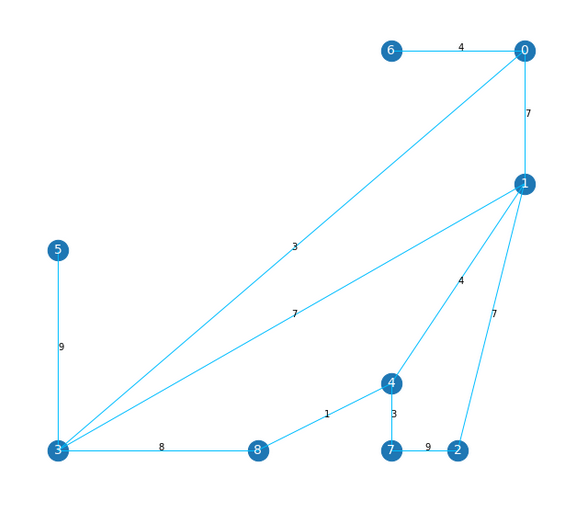Consider the following graph G. It consist of 9 nodes labeled with numbers as well as 12 edges connecting the nodes. Each edge is labeled with its cost. Let 2 be the start node and 8 be the goal node. Run Dijkstra’s algorithm on G. What is the total cost of the path from start to goal?Consider the following graph G. It consist of 9 nodes labeled with numbers as well as 12 edges connecting the nodes. Each edge is labeled with its cost. Let 7 be the start node and 5 be the goal node. Run Dijkstra’s algorithm on G. What is the total cost of the path from start to goal?Consider the following graph G. It consist of 9 nodes labeled with numbers as well as 12 edges connecting the nodes. Each edge is labeled with its cost. Let 6 be the start node and 7 be the goal node. Run Dijkstra’s algorithm on G. What is the total cost of the path from start to goal?Consider the following graph G. It consist of 9 nodes labeled with numbers as well as 12 edges connecting the nodes. Each edge is labeled with its cost. Let 5 be the start node and 7 be the goal node. Run Dijkstra’s algorithm on G. What is the total cost of the path from start to goal?Consider the following graph G. It consist of 9 nodes labeled with numbers as well as 12 edges connecting the nodes. Each edge is labeled with its cost. Let 0 be the start node and 7 be the goal node. Run Dijkstra’s algorithm on G. What is the total cost of the path from start to goal?Consider the following graph G. It consist of 9 nodes labeled with numbers as well as 12 edges connecting the nodes. Each edge is labeled with its cost. Let 2 be the start node and 5 be the goal node. Run Dijkstra’s algorithm on G. What is the total cost of the path from start to goal?Consider the following graph G. It consist of 9 nodes labeled with numbers as well as 12 edges connecting the nodes. Each edge is labeled with its cost. Let 6 be the start node and 8 be the goal node. Run Dijkstra’s algorithm on G. What is the total cost of the path from start to goal?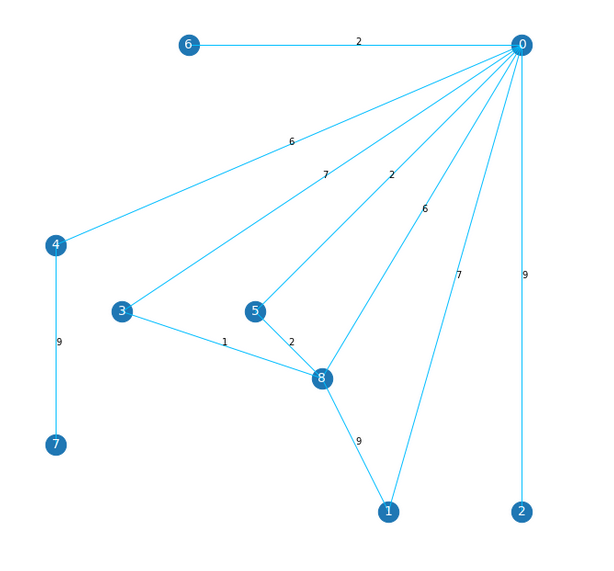Consider the following graph G. It consist of 9 nodes labeled with numbers as well as 12 edges connecting the nodes. Each edge is labeled with its cost. Let 7 be the start node and 3 be the goal node. Run Dijkstra’s algorithm on G. What is the total cost of the path from start to goal?Consider the following graph G. It consist of 9 nodes labeled with numbers as well as 12 edges connecting the nodes. Each edge is labeled with its cost. Let 5 be the start node and 7 be the goal node. Run Dijkstra’s algorithm on G. What is the total cost of the path from start to goal?Consider the following graph G. It consist of 9 nodes labeled with numbers as well as 12 edges connecting the nodes. Each edge is labeled with its cost. Let 4 be the start node and 3 be the goal node. Run Dijkstra’s algorithm on G. What is the total cost of the path from start to goal?Consider the following graph G. It consist of 9 nodes labeled with numbers as well as 12 edges connecting the nodes. Each edge is labeled with its cost. Let 2 be the start node and 3 be the goal node. Run Dijkstra’s algorithm on G. What is the total cost of the path from start to goal?Consider the following graph G. It consist of 9 nodes labeled with numbers as well as 12 edges connecting the nodes. Each edge is labeled with its cost. Let 3 be the start node and 6 be the goal node. Run Dijkstra’s algorithm on G. What is the total cost of the path from start to goal?Consider the following graph G. It consist of 9 nodes labeled with numbers as well as 12 edges connecting the nodes. Each edge is labeled with its cost. Let 6 be the start node and 3 be the goal node. Run Dijkstra’s algorithm on G. What is the total cost of the path from start to goal?Consider the following graph G. It consist of 9 nodes labeled with numbers as well as 12 edges connecting the nodes. Each edge is labeled with its cost. Let 3 be the start node and 7 be the goal node. Run Dijkstra’s algorithm on G. What is the total cost of the path from start to goal?Consider the following graph G. It consist of 9 nodes labeled with numbers as well as 12 edges connecting the nodes. Each edge is labeled with its cost. Let 8 be the start node and 7 be the goal node. Run Dijkstra’s algorithm on G. What is the total cost of the path from start to goal?Consider the following graph G. It consist of 9 nodes labeled with numbers as well as 12 edges connecting the nodes. Each edge is labeled with its cost. Let 7 be the start node and 1 be the goal node. Run Dijkstra’s algorithm on G. What is the total cost of the path from start to goal?Consider the following graph G. It consist of 9 nodes labeled with numbers as well as 12 edges connecting the nodes. Each edge is labeled with its cost. Let 2 be the start node and 8 be the goal node. Run Dijkstra’s algorithm on G. What is the total cost of the path from start to goal?Consider the following graph G. It consist of 9 nodes labeled with numbers as well as 12 edges connecting the nodes. Each edge is labeled with its cost. Let 5 be the start node and 7 be the goal node. Run Dijkstra’s algorithm on G. What is the total cost of the path from start to goal?Consider the following graph G. It consist of 9 nodes labeled with numbers as well as 12 edges connecting the nodes. Each edge is labeled with its cost. Let 8 be the start node and 4 be the goal node. Run Dijkstra’s algorithm on G. What is the total cost of the path from start to goal?Consider the following graph G. It consist of 9 nodes labeled with numbers as well as 12 edges connecting the nodes. Each edge is labeled with its cost. Let 2 be the start node and 7 be the goal node. Run Dijkstra’s algorithm on G. What is the total cost of the path from start to goal?Consider the following graph G. It consist of 9 nodes labeled with numbers as well as 12 edges connecting the nodes. Each edge is labeled with its cost. Let 8 be the start node and 6 be the goal node. Run Dijkstra’s algorithm on G. What is the total cost of the path from start to goal?Consider the following graph G. It consist of 9 nodes labeled with numbers as well as 12 edges connecting the nodes. Each edge is labeled with its cost. Let 6 be the start node and 8 be the goal node. Run Dijkstra’s algorithm on G. What is the total cost of the path from start to goal?Consider the following graph G. It consist of 9 nodes labeled with numbers as well as 12 edges connecting the nodes. Each edge is labeled with its cost. Let 7 be the start node and 8 be the goal node. Run Dijkstra’s algorithm on G. What is the total cost of the path from start to goal?Consider the following graph G. It consist of 9 nodes labeled with numbers as well as 12 edges connecting the nodes. Each edge is labeled with its cost. Let 2 be the start node and 8 be the goal node. Run Dijkstra’s algorithm on G. What is the total cost of the path from start to goal?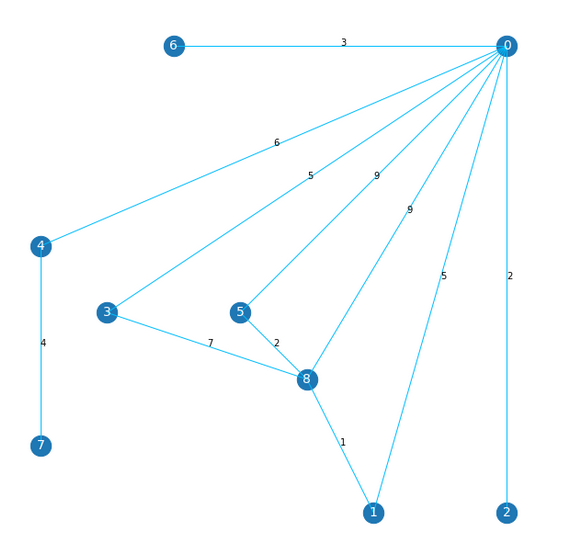Consider the following graph G. It consist of 9 nodes labeled with numbers as well as 12 edges connecting the nodes. Each edge is labeled with its cost. Let 7 be the start node and 8 be the goal node. Run Dijkstra’s algorithm on G. What is the total cost of the path from start to goal?Consider the following graph G. It consist of 9 nodes labeled with numbers as well as 12 edges connecting the nodes. Each edge is labeled with its cost. Let 4 be the start node and 8 be the goal node. Run Dijkstra’s algorithm on G. What is the total cost of the path from start to goal?Consider the following graph G. It consist of 9 nodes labeled with numbers as well as 12 edges connecting the nodes. Each edge is labeled with its cost. Let 5 be the start node and 7 be the goal node. Run Dijkstra’s algorithm on G. What is the total cost of the path from start to goal?Consider the following graph G. It consist of 9 nodes labeled with numbers as well as 12 edges connecting the nodes. Each edge is labeled with its cost. Let 7 be the start node and 0 be the goal node. Run Dijkstra’s algorithm on G. What is the total cost of the path from start to goal?Consider the following graph G. It consist of 9 nodes labeled with numbers as well as 12 edges connecting the nodes. Each edge is labeled with its cost. Let 1 be the start node and 6 be the goal node. Run Dijkstra’s algorithm on G. What is the total cost of the path from start to goal?Consider the following graph G. It consist of 9 nodes labeled with numbers as well as 12 edges connecting the nodes. Each edge is labeled with its cost. Let 6 be the start node and 7 be the goal node. Run Dijkstra’s algorithm on G. What is the total cost of the path from start to goal?Consider the following graph G. It consist of 9 nodes labeled with numbers as well as 12 edges connecting the nodes. Each edge is labeled with its cost. Let 1 be the start node and 4 be the goal node. Run Dijkstra’s algorithm on G. What is the total cost of the path from start to goal?Consider the following graph G. It consist of 9 nodes labeled with numbers as well as 12 edges connecting the nodes. Each edge is labeled with its cost. Let 8 be the start node and 6 be the goal node. Run Dijkstra’s algorithm on G. What is the total cost of the path from start to goal?Consider the following graph G. It consist of 9 nodes labeled with numbers as well as 12 edges connecting the nodes. Each edge is labeled with its cost. Let 6 be the start node and 8 be the goal node. Run Dijkstra’s algorithm on G. What is the total cost of the path from start to goal?Consider the following graph G. It consist of 9 nodes labeled with numbers as well as 12 edges connecting the nodes. Each edge is labeled with its cost. Let 7 be the start node and 6 be the goal node. Run Dijkstra’s algorithm on G. What is the total cost of the path from start to goal?Consider the following graph G. It consist of 9 nodes labeled with numbers as well as 12 edges connecting the nodes. Each edge is labeled with its cost. Let 4 be the start node and 1 be the goal node. Run Dijkstra’s algorithm on G. What is the total cost of the path from start to goal?Consider the following graph G. It consist of 9 nodes labeled with numbers as well as 12 edges connecting the nodes. Each edge is labeled with its cost. Let 6 be the start node and 1 be the goal node. Run Dijkstra’s algorithm on G. What is the total cost of the path from start to goal?Consider the following graph G. It consist of 9 nodes labeled with numbers as well as 12 edges connecting the nodes. Each edge is labeled with its cost. Let 4 be the start node and 8 be the goal node. Run Dijkstra’s algorithm on G. What is the total cost of the path from start to goal?Consider the following graph G. It consist of 9 nodes labeled with numbers as well as 12 edges connecting the nodes. Each edge is labeled with its cost. Let 5 be the start node and 7 be the goal node. Run Dijkstra’s algorithm on G. What is the total cost of the path from start to goal?Consider the following graph G. It consist of 9 nodes labeled with numbers as well as 12 edges connecting the nodes. Each edge is labeled with its cost. Let 4 be the start node and 7 be the goal node. Run Dijkstra’s algorithm on G. What is the total cost of the path from start to goal?Consider the following graph G. It consist of 9 nodes labeled with numbers as well as 12 edges connecting the nodes. Each edge is labeled with its cost. Let 8 be the start node and 4 be the goal node. Run Dijkstra’s algorithm on G. What is the total cost of the path from start to goal?Consider the following graph G. It consist of 9 nodes labeled with numbers as well as 12 edges connecting the nodes. Each edge is labeled with its cost. Let 6 be the start node and 1 be the goal node. Run Dijkstra’s algorithm on G. What is the total cost of the path from start to goal?Consider the following graph G. It consist of 9 nodes labeled with numbers as well as 12 edges connecting the nodes. Each edge is labeled with its cost. Let 6 be the start node and 5 be the goal node. Run Dijkstra’s algorithm on G. What is the total cost of the path from start to goal?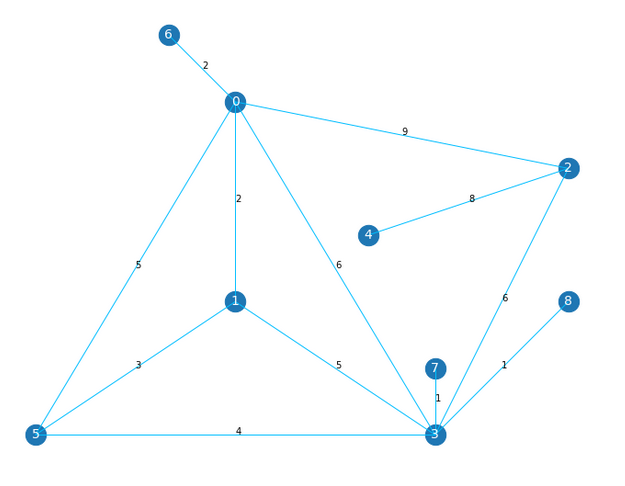Consider the following graph G. It consist of 9 nodes labeled with numbers as well as 12 edges connecting the nodes. Each edge is labeled with its cost. Let 5 be the start node and 4 be the goal node. Run Dijkstra’s algorithm on G. What is the total cost of the path from start to goal?Consider the following graph G. It consist of 9 nodes labeled with numbers as well as 12 edges connecting the nodes. Each edge is labeled with its cost. Let 4 be the start node and 1 be the goal node. Run Dijkstra’s algorithm on G. What is the total cost of the path from start to goal?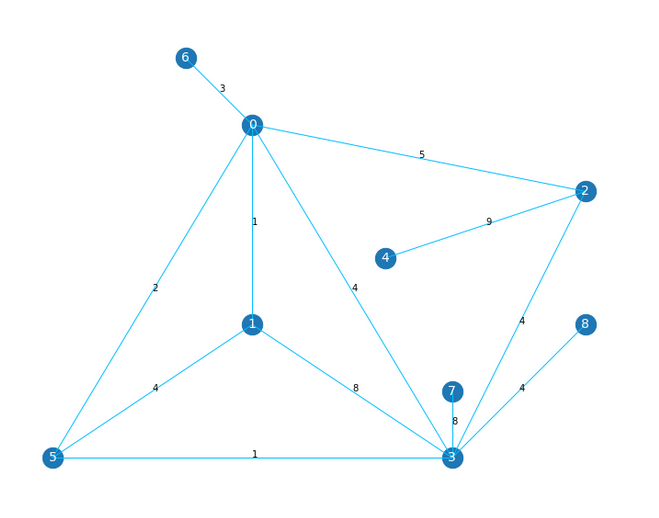Consider the following graph G. It consist of 9 nodes labeled with numbers as well as 12 edges connecting the nodes. Each edge is labeled with its cost. Let 8 be the start node and 6 be the goal node. Run Dijkstra’s algorithm on G. What is the total cost of the path from start to goal?Consider the following graph G. It consist of 9 nodes labeled with numbers as well as 12 edges connecting the nodes. Each edge is labeled with its cost. Let 7 be the start node and 6 be the goal node. Run Dijkstra’s algorithm on G. What is the total cost of the path from start to goal?Consider the following graph G. It consist of 9 nodes labeled with numbers as well as 12 edges connecting the nodes. Each edge is labeled with its cost. Let 6 be the start node and 8 be the goal node. Run Dijkstra’s algorithm on G. What is the total cost of the path from start to goal?

Posting submission...